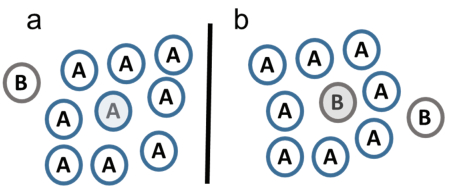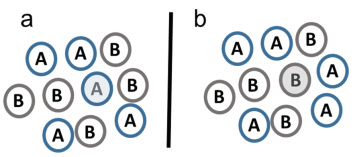## Relationship and Difference among Raoult's Law, Henry's Law, and Azeotropic Solution

 基金资助: 国家自然科学基金.  21375064国家自然科学基金.  21575090国家重点基础研究发展计划（973计划）.  2015CB932001

 Fund supported: 国家自然科学基金.  21375064国家自然科学基金.  21575090国家重点基础研究发展计划（973计划）.  2015CB932001Abstract

Gas-liquid equilibrium is important in classroom teaching and practical production. In this paper, the relationship and difference among Raoult's Law, Henry's Law, and azeotropic solution are discussed from the point of microscopic view. The different interactions between solvent-solvent, solvent-solute, and solute-solute lead to that the two laws are only useful for dilute solution. When the apparent vapor pressure of the solvent and solute are equal to each other by tuning their mole fraction, the azeotropic solution is produced.

Keywords： Raoult's Law ; Henry's Law ; Azeotropic solution ; Microscopic view

YIN Xuebo. Relationship and Difference among Raoult's Law, Henry's Law, and Azeotropic Solution. University Chemistry[J], 2018, 33(5): 61-65 doi:10.3866/PKU.DXHX201801010

## 1 拉乌尔定律和亨利定律的微观解释

${{p}_{\operatorname{A}}}=p_{\text{A}}^{*}\times {{x}_{\text{A}}}$

${{p}_{\text{B}}}={{K}_{\text{B}}}\times {{x}_{\text{B}}}$

${{p}_{\text{B}}}={{K}_{\text{m, B}}}\times {{m}_{\text{B}}}$

${{p}_{\text{B}}}={{K}_{\text{c, B}}}\times {{c}_{\text{B}}}$

### 图1### 图2(a)加入B物质导致A物质的表观蒸气压大于其饱和蒸气压；(b)加入B物质导致A物质的表观蒸气压小于其饱和蒸气压

## 2 恒沸溶液

$\frac{{{n}_{\text{A, l}}}}{{{n}_{\text{B, l}}}}=\frac{{{n}_{\text{A, g}}}}{{{n}_{\text{B, g}}}}$

$\frac{{{n}_{\text{A,l}}}}{{{n}_{\text{B,l}}}}=\frac{{{n}_{\text{A,g}}}}{{{n}_{\text{B,g}}}}=\frac{{{p}_{\text{A}}}}{{{p}_{\text{B}}}}=\frac{{{{{K}'}}_{\text{A}}}\times {{x}_{\text{A,l}}}}{{{{{K}'}}_{\text{B}}}\times {{x}_{\text{B,l}}}}=\frac{{{{{K}'}}_{\text{A}}}\times \frac{{{n}_{\text{A,l}}}}{{{n}_{\text{A,l}}}+{{n}_{\text{B,l}}}}}{{{{{K}'}}_{\text{B}}}\times \frac{{{n}_{\text{B,l}}}}{{{n}_{\text{A,l}}}+{{n}_{\text{B,l}}}}}=\frac{{{{{K}'}}_{\text{A}}}\times {{n}_{\text{A,l}}}}{{{{{K}'}}_{\text{B}}}\times {{n}_{\text{B,l}}}}$

### 图3## 参考文献 原文顺序 文献年度倒序 文中引用次数倒序 被引期刊影响因子

Kugel R. W. J. Chem. Educ. 1998, 75 (9), 1125.

Horowitz G. ; Viswanathan R. J. Chem. Educ. 1998, 75 (9), 1124.

/

 〈〉